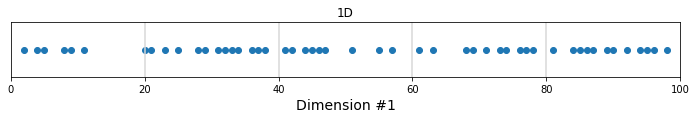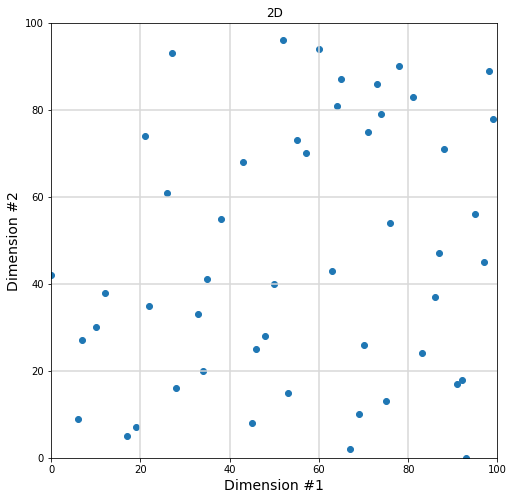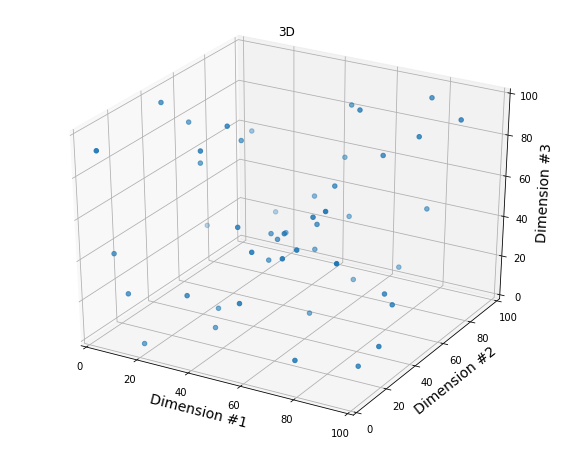# Artificial Intelligence

## The Curse of Dimensionality – Illustrated With Matplotlib

Maybe you already came across this famous quote in Machine Learning by Charles Lee Isbell

“As the number of features or dimensions grows, the amount of data we need to generalize accurately grows exponentially.”

Here is another explanation from Wikipedia

“When the dimensionality increases, the volume of the space increases so fast that the available data become sparse. (…) In order to obtain a statistically sound and reliable result, the amount of data needed to support the result often grows exponentially with the dimensionality.”

I think the  “Curse of Dimensionality” is easier to understand when visualized. Suppose you have 50 data points between 0 and 100.

### 1- Let’s try with one dimension first

```import pandas as pd
import matplotlib.pyplot as plt
import random
import numpy as np

fig = plt.figure()
ax  = plt.axes()
fig.set_size_inches(12, 1)

x = random.sample(range(0, 100), 50)
y = [0 for xval in x]
plt.scatter(x, y)

# Grid lines
for grid_pt in [20, 40, 60, 80]:
plt.axvline(x=grid_pt, color='#D8D8D8')

ax.set_xlim((0,100))
ax.set_xlabel("Dimension #1", fontsize=14)
ax.set_ylabel("")
plt.yticks([], [])
plt.title("1D")
plt.show()```With 5 intervals in our first dimension, there will be an average of 50/5 = 10 points per cell, which is already low if you’d like to do any statistical analysis for each interval.

### 2- Moving to two dimensions

```fig = plt.figure()
ax  = plt.axes()
fig.set_size_inches(8, 8)

# Now each point has 2 dimensions (x,y)
x = random.sample(range(0, 100), 50)
y = random.sample(range(0, 100), 50)

plt.scatter(x, y)

# Grid lines
for grid_pt in [20, 40, 60, 80]:
plt.axvline(x=grid_pt, color='#D8D8D8')
plt.axhline(y=grid_pt, color='#D8D8D8')

ax.set_xlim((0,100))
ax.set_ylim((0,100))
ax.set_xlabel("Dimension #1", fontsize=14)
ax.set_ylabel("Dimension #2", fontsize=14)
plt.title("2D")
plt.show()```With 5 intervals on the first dimension and 5 intervals on the 2nd dimension, we now have 50/(5×5) = 2 points per cell on average. In fact, we are already starting to see cells that do not have any data to work with.

### 3- Adding a third dimension

```from mpl_toolkits import mplot3d

fig = plt.figure()
fig.set_size_inches(10, 8)

# Now each point has 3 dimensions (x,y,x)
x = random.sample(range(0, 100), 50)
y = random.sample(range(0, 100), 50)
z = random.sample(range(0, 100), 50)

ax.scatter(x, y, z)

# Grid lines
for grid_pt in [20, 40, 60, 80]:
plt.axvline(x=grid_pt, color='#D8D8D8')
plt.axhline(y=grid_pt, color='#D8D8D8')

ax.set_xlim(0,100)
ax.set_ylim(0,100)
ax.set_zlim(0,100)

ax.set_xlabel("Dimension #1", fontsize=14)
ax.set_ylabel("Dimension #2", fontsize=14)
ax.set_zlabel("Dimension #3", fontsize=14)
plt.title("3D")
plt.show()```With 5 intervals on the third dimension, we have 50/(5x5x5) = 0.4 points per cell on average!

### In Conclusion

As you add new dimensions, you create “new space” that is usually not filled properly by your initial data.

In order to obtain a statistically sound and reliable result, the amount of data needed to support the result often grows exponentially with the dimensionality.

## Visualize a Decision Tree with Sklearn

##### Step 1: Install the libraries
```sudo apt-get install graphviz

pip install graphviz
pip install pydotplus
pip install sklearnpip install pydot
pip install pandas```

Do the imports

```import pydotplus
import pandas as pd
from sklearn import tree
from io import StringIO
import pydot
```
##### Step 2: Initialize the dataframe
```data = [
(0, 5, 0),
(1, 6, 0),
(2, 7, 1),
(3, 8, 1),
(4, 9, 1)
]
df = pd.DataFrame(data, index=range(5), columns=['x1','x2','y'])```
##### Step 3: Train the decision tree
```x_columns = ['x1','x2']

model = tree.DecisionTreeClassifier()
trained_model = model.fit(df[x_columns], df['y'])
```
##### Step 4: Display the decision tree

Two options

Option A: You want to save the decision tree as a file

```dotfile = StringIO()

tree.export_graphviz(
trained_model,
out_file        = dotfile,
feature_names   = x_columns,
class_names     = ['[y=0]', '[y=1]'], # Ascending numerical order
filled          = True,
rounded         = True
)

(graph,) = pydot.graph_from_dot_data(dotfile.getvalue())
graph.write_png("tree.png")```

This should generate an image named “tree.png” in your current directory

Option B: You want to display the decision tree in your Jupyter notebook

```from IPython.display import Image

out_file = tree.export_graphviz(
trained_model,
feature_names   = x_columns,
class_names     = ['[y=0]', '[y=1]'],# Ascending numerical order
filled          = True,
rounded         = True
)
graph = pydotplus.graph_from_dot_data(out_file)
Image(graph.create_png())
```

In either case this is the tree you should getReferences:

https://scikit-learn.org/stable/modules/generated/sklearn.tree.export_graphviz.html